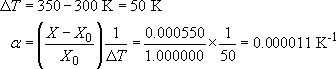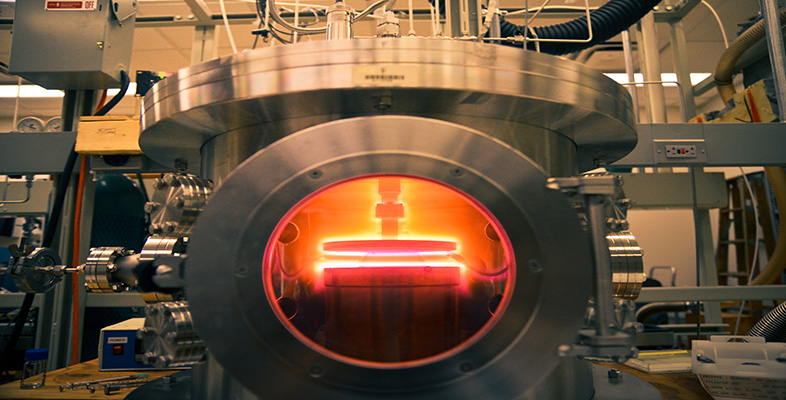Engineering: The challenge of temperature

Start this free course now. Just create an account and sign in. Enrol and complete the course for a free statement of participation or digital badge if available.

Free course

# Engineering: The challenge of temperature

## 3.1 Modelling properties

This section provides a model for properties interpreted in terms of the average thermal energy of all the constituent atoms of a material. Since absolute temperature T is a measure of average atomic kinetic energy, we shall expect to be looking at properties that change gradually with T, roughly proportionally, over a wide range. In terms of the classification introduced in Figure 3, we shall be looking at changes like Figure 3(a). The experimental descriptions of such changes usually use power series, setting the property X, for example the length of a specimen, as a function of a change in temperature, ΔT (here the Greek capital letter delta, Δ, is used to express a change in the quantity that follows it):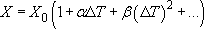Often the constant β is small enough that ΔX is almost proportional to ΔT. Then α is called the linear temperature coefficient of the property (expansion, resistance or whatever) and we can express the change in X as: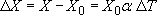In particular, I want to discuss details of the thermal expansion of solids, but the temperature dependence of the electrical resistance of metals, the thermal conductivity of solids and the Young's modulus of many materials also vary in a gradual way.

### Exercise 4

The length of a steel rod is precisely measured to be 1.000000 m at 300 K. When heated to 350 K it is measured again and found to be 1.000550 m. Assuming the change to be linear, calculate the coefficient α.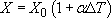The change in length of the steel rod is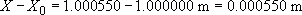The change in temperature is